# Team:AUC TURKEY/Project/ModellingA KINETIC MODEL IN MATLAB

How did we build it???

Using the SimBiology toolbox for MATLAB we created a framework of the enzyme kinetic modelling(Figure 1).

HRP + H2O2 → ES1 (HRP compound I) + MG → ES2(MGox +HRP compound II)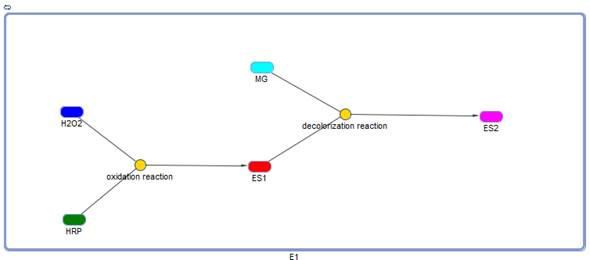After creating the basic framework for the model we needed to create mathematical equations for each reaction with appropriate rate constants. These equations and the corresponding values are shown below in Tables 1 and 2.

 Reaction Name: Reaction Scheme: Reaction Rate: oxidation reaction H2O2 + HRP -> ES1 k1*H2O2*HRP decolourization reaction ES1 + MG -> ES2 k2*ES1*MG

Table1: Mathematical representation of enzyme kinetic reaction expression and the subsequent production of the oxidized dye. Constants are described in Table 2.

 Constant: Value: Units: References: k1 0.0018 1/(µmolarity*min) Viridiana S. Ferreira-Leitão (2002) k1r neglected - k2 0.004 1/(µmolarity*min) Viridiana S. Ferreira-Leitão (2002) k2r neglected -

Table 2: Values assigned to kinetic parameters described in Table 1. We ignored k1r and k2r values because of k1>>>>k1r and k2>>>k2r, so our both reactions act as irreversible.

 Rule Name: Rate Rules: HRP dE/dt = - k1[E][S1]+k1r[ES1] H2O2 dS1/dt = -k1[E][S1]+k1r[ES1] ES1 (HRP compound I) dES1/dt =k1[E][S1] –k1r[ES1]-k2[ES1][S2]+k2r[ES2] Methyl Green (MG) dS2/dt = -k2[ES1][S2] +k2r[ES2] ES2 (MGox +LiP compound II) dES2/dt = k2[ES1][S2] – k2r[ES2]

Table3: Differential equations of reactions.

What did it show???

Before running the model we needed to decide what an appropriate endpoint of reaction would be. Therefore, concentrations of species were determined from the literature and necessary assumptions were made to check our cascade reactions work correctly.

According to chosen references points, we were trying to determine how quickly we could get a decolourization. Decolourization depends on final [ES2] (MGox +HRP compound II) concentration and degradation of MB.

Decolourization starts in 40 minutes.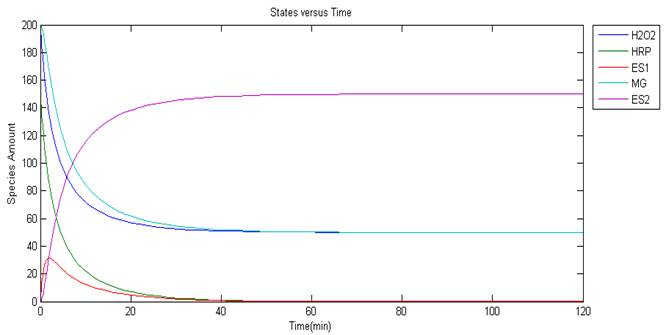Reaction Name: Reaction Scheme: Reaction Rate: oxidation reaction H2O2 + HRP -> ES1 k1*H2O2*HRP decolorization reaction ES1 + MB -> ES2 k2*ES1*MB

Table 4: Mathematical representation of enzyme kinetic reaction expression and the subsequent production of the oxidized dye. Constants are described in Table 5.

 Constant: Value: Units: References: k1 0.0018 1/(µmolarity*min) Viridiana S. Ferreira-Leitão (2002) k1r neglected - k2 0.004 1/(µmolarity*min) Viridiana S. Ferreira-Leitão (2002) k2r neglected -

Table 5: Values assigned to kinetic parameters described in Table 4. We ignored k1r and k2r values because of k1>>>>k1r and k2>>>k2r, so our both reactions act as irreversible.

 Rule Name: Rate Rules: HRP dE/dt = - k1[E][S1]+k1r[ES1] H2O2 dS1/dt = -k1[E][S1]+k1r[ES1] ES1 (HRP compound I) dES1/dt =k1[E][S1] –k1r[ES1]-k2[ES1][S2]+k2r[ES2] Methylen Blue (MB) dS2/dt = -k2[ES1][S2] +k2r[ES2] ES2 (MBox +LiP compound II) dES2/dt = k2[ES1][S2] – k2r[ES2]

Table6: Differential equations of reactions.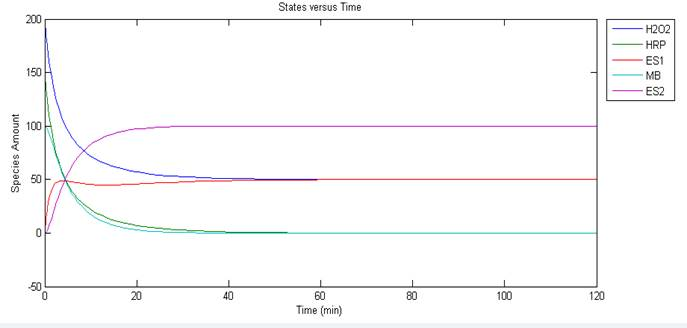1hour of HRP- MB decolourization

What did it show???

Before running the model we needed to decide what an appropriate endpoint of reaction would be. Therefore, concentrations of species were determined from the literature and necessary assumptions were made to check our cascade reactions work correctly.

According to chosen references points, we were trying to determine how quickly we could get a decolourization. Decolourization depends on final [ES2] (MBox +LiP compound II) concentration and degradation of MB.

Decolourization starts in 40 minutes.

LiP + H2O2 →ES1 (LiP compound I ) + MB → ES2 (MBox +LiP compound II)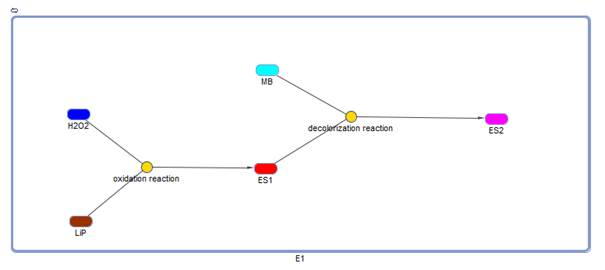Reaction Name: Reaction Scheme: Reaction Rate: oxidation reaction H2O2 + LiP -> ES1 k1*H2O2*LiP decolorization reaction ES1 + MB -> ES2 k2*ES1*MB

Table7: Mathematical representation of enzyme kinetic reaction expression and the subsequent production of the oxidized dye. Constants are described in Table 8.

 Constant: Value: Units: References: k1 1.0E-4 1/(µmolarity*min) Paulı Ollıkka, Kırsı Alhonmakı (1993) k1r neglected - k2 2.0E-4 1/(µmolarity*min) Paulı Ollıkka, Kırsı Alhonmakı (1993) k2r neglected -

Table 8: Values assigned to kinetic parameters described in Table 7. We ignored k1r and k2r values because of k1>>>>k1r and k2>>>k2r, so our both reactions act as irreversible.

 Rule Name: Rate Rules: HRP dE/dt = - k1[E][S1]+k1r[ES1] H2O2 dS1/dt = -k1[E][S1]+k1r[ES1] ES1 (HRP compound I) dES1/dt =k1[E][S1] –k1r[ES1]-k2[ES1][S2]+k2r[ES2] Methylen Blue (MB) dS2/dt = -k2[ES1][S2] +k2r[ES2] ES2 (MBox +LiP compound II) dES2/dt = k2[ES1][S2] – k2r[ES2]

Table9: Differential equations of reactions.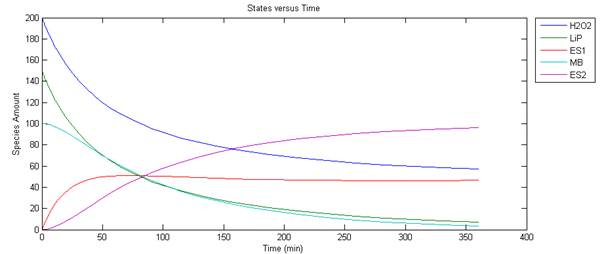6 hours of Lip- MG decolourization

What did it show???

Before running the model we needed to decide what an appropriate endpoint of reaction would be. Therefore, concentrations of species were determined from the literature and necessary assumptions were made to check our cascade reactions work correctly.

According to chosen references points, we were trying to determine how quickly we could get a decolourization. Decolourization depends on final [ES2] (Mgox +Lip compound II) concentration and degradation of MG.

Decolourization starts in 40 minutes.

 Reaction Name: Reaction Scheme: Reaction Rate: oxidation reaction H2O2 + LiP -> ES1 k1*H2O2*LiP decolourization reaction ES1 + MG -> ES2 k2*ES1*MG

Table10: Mathematical representation of enzyme kinetic reaction expression and the subsequent production of the oxidized dye. Constants are described in Table 11.

 Constant: Value: Units: References: k1 1.0E-4 1/(µmolarity*min) Paulı Ollıkka, Kırsı Alhonmakı (1993) k1r neglected - k2 2.0E-4 1/(µmolarity*min) Paulı Ollıkka, Kırsı Alhonmakı (1993) k2r neglected -

Table 11: Values assigned to kinetic parameters described in Table 10. We ignored k1r and k2r values because of k1>>>>k1r and k2>>>k2r, so our both reactions act as irreversible

 Rule Name: Rate Rules: HRP dE/dt = - k1[E][S1]+k1r[ES1] H2O2 dS1/dt = -k1[E][S1]+k1r[ES1] ES1 (HRP compound I) dES1/dt =k1[E][S1] –k1r[ES1]-k2[ES1][S2]+k2r[ES2] Methyl Green (MG) dS2/dt = -k2[ES1][S2] +k2r[ES2] ES2 (MGox +LiP compound II) dES2/dt = k2[ES1][S2] – k2r[ES2]

Table12: Differential equations of reactions.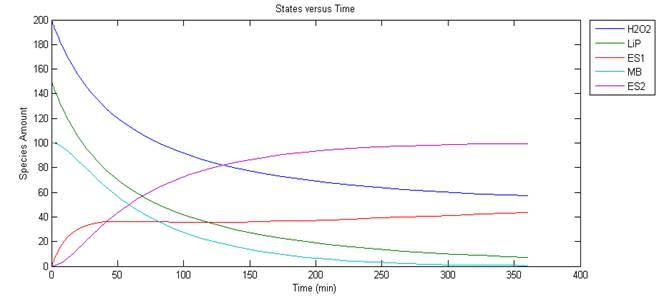LiP – MB 5 hours of decolorization

What did it show???

Before running the model we needed to decide what an appropriate endpoint of reaction would be. Therefore, concentrations of species were determined from the literature and necessary assumptions were made to check our cascade reactions work correctly.

According to chosen references points, we were trying to determine how quickly we could get a decolourization. Decolourization depends on final [ES2] (MBox +Lip compound II) concentration and degradation of MB.

Decolourization starts in 40 minutes.

Future plans

The most important results that we were able to achieve were the correlation between the functional assays the modelling results of our MATLAB kinetic model. In the modelling, the decolourizations start at the approximately 40 minutes while these are 60 minutes for the assay. These differences were due to the consideration of the used HRP and LIP enzymes in the modelling as purified allowing more direct interaction in between the hydrogen peroxide and the dyes. The assay was not conducted with purified enzymes but with lysate acquired from liquid culture containing impurities which slow down the conduction of the reaction. In the future, we hope to extract the enzymes from the bacteria to allow the direct contact of the enzymes and the dyes to increase spontaneity.

Thanks to Ayşe Çelik for her support.